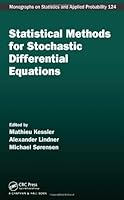# Statistical Methods for Stochastic Differential Equations## Book Description

Statistical Methods for Stochastic Differential Equations (Chapman & Hall/CRC Monographs on & Applied Probability)

The seventh volume in the SemStat series, Statistical Methods for Stochastic Differential Equations presents current research trends and recent developments in statistical methods for stochastic differential equations. Written to be accessible to both new students and seasoned researchers, each self-contained chapter starts with introductions to the topic at hand and builds gradually towards discussing recent research.

The book covers Wiener-driven equations as well as stochastic differential equations with jumps, including continuous-time ARMA processes and COGARCH processes. It presents a spectrum of estimation methods, including nonparametric estimation as well as parametric estimation based on likelihood methods, estimating , and simulation techniques. Two chapters are devoted to high-frequency data. Multivariate are also considered, including partially observed , asynchronous sampling, tests for simultaneous jumps, and multiscale diffusions.

Statistical Methods for Stochastic Differential Equations is useful to the theoretical statistician and the probabilist who works in or intends to work in the field, as well as to the applied statistician or econometrician who needs the methods to analyze biological or time series.

Chapter 1. Estimating functions for diffusion-type processes
Chapter 2. The econometrics of high-frequency data
Chapter 3. Statistics and high-frequency data
Chapter 4. Importance sampling techniques for estimation of diffusion models
Chapter 5. Non-parametric estimation of the coefficients of ergodic diffusion processes based on high-frequency data
Chapter 6. Ornstein–Uhlenbeck related models driven by Lévy processes
Chapter 7. Parameter estimation for multiscale diffusions: An overview